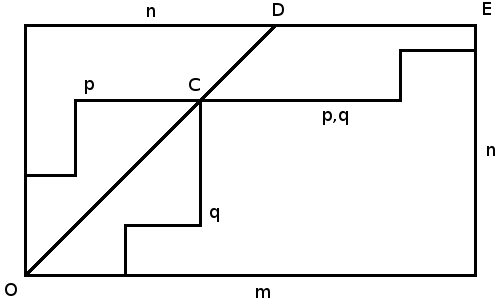Want to share your content on R-bloggers? click here if you have a blog, or here if you don't.This year, for Halloween, a post on candies (I promise, next year I will write another post on zombies). But I don’t want to focus on the kids problems (last year, we tried to minimize their walking distance to collect as much candies as possible, with part 1 and part 2), I want to discuss my own problems. Because usually, the kids wear their costumes, and they go in the streets,they knock on the doors, while I stay at home. So I’m the one, with a bag full of candies, waiting for kids to knock on our door, and then I give them some candies (if they wear a costume). Consider the following problem. Assume that we start with  red candies, and  black ones, with . The thing is that no one like those black candies. What could be the probability that for the   kids that will get candies after knocking at my door (with  for convenience, but we will also consider the more general case where I have to many candies, , later on), the probability to get a red candy is always larger than the probability to have a black candy ? This is somehow related to the popular ballot problem, proposed (and solved) by Whitworth in 1878, but he wrote it only in the fourth edition of Choice and Chance, in 1886 (this is what the legend told us). In 1887, Joseph Bertrand proposed a similar problem, and Désiré André introduced the reflection principle to solve it. The problem is simple : consider an election between two candidates, A (who receives  votes) and B (who receives  votes). A wins the election (). If the ballots are cast one at a time, what is the probability that A will lead throughout the voting? For those who don’t remember the conclusion, the probability is here quite simple,

Observe that some geometry proofs were given, later on, by Aebly or Mirimanoff, both in 1923, as well as Howard Grossman in the 1950′s (see the discussion on http://academiclogbook.blogspot.ca/…).  Actually, http://futilitycloset.com// produced the following geometric proof (with no clear reference),We start at O, where no votes have been cast. Each vote for A moves us one point east and each vote for B moves us one point north until we arrive at E, the final count, (mn). If A is to lead throughout the contest, then our path must steer consistently east of the diagonal line OD, which represents a tie score. Any path that starts by going north, through (0,1), must cut OD on its way to E.

If any path does touch OD, let it be at C. The group of such paths can be paired off as p and q, reflections of each other in the line OD that meet at C and continue on a common track to E.

This means that the total number of paths that touch OD is twice the number of paths p that start their journey to E by going north. Now, the first segment of any path might be up to m units east or up to units north, so the proportion of paths that start by going north is n/(m + n), and twice this number is 2n/(m + n). The complementary probability — the probability of a path not touching OD — is (m –n)/(m + n).

But let’s try to solve our problem. Let  and  denote the number of black and red candies, respectively after the th kid git his (or her) candy. Yes, one at a time. Here,  and . What we want is

Using this formulation, we recognize the ballot problem. Almost. Actually, in the original ballot problem (see Bertrand (1887)), we have to compute the probability that one candidate remains strictly ahead the other one throughout the count. With a strict condition, we get the well-known probability (given previously)

Here, ties are allowed, and we can prove (easily) that

(again, there is some nice geometric interpenetration of that result). It is also possible to get numerically that value using the following function, which will generate a trajectory, and return some indicators (with or without ties)

```> red_black=function(sd){
+ set.seed(sd)
+ vectcandy=sample(c(rep("R",r),rep("B",b)))
+ v1=rev(cumsum(rev(vectcandy)=="R"))<rev(cumsum(rev(vectcandy)=="B"))
+ v2=cumsum(rev(vectcandy)=="R")<= cumsum(rev(vectcandy)=="B")
+ return(list(evol=cbind(rev(cumsum(rev(vectcandy)=="R")),
+ rev(cumsum(rev(vectcandy)=="B")),v1),list=vectcandy,test=(sum(v1)==0),
+ ballot=(sum(v2)==0),when=min(which(v1==1))))}```

(here I compute the ballot-type index, where ties are not allowed, and the candy-type index). If we generate 100,000 scenarios, starting with 50 red and 25 black candies, we get

```> r=50
> b=25
> M=sapply(1:100000,red_black) ­­
> mean(unlist(M[3,]))
 0.50967```

which can be compared with the theoretical value

```> (r+1-b)/(r+1)
 0.5098039```

We can also get the distribution of the first time we have more black candies than red ones left (given that this event occur)

```> Z=unlist(M[5,])
> Z=Z[Z<Inf]
> hist(Z,breaks=seq(0,80),probability=TRUE,col="light blue",
+ border=NA,xlab="",main="")```

There might be some analytically formula that can be derived, but I have to confess that I am becoming extremely lazy,Assume now that this year, kids do not show up at my door (for some reason). Assume that  kids show up. We can see how the probability

will change, with ,

```> r=50
> b=25
> impact_n = function(n){
+ red_black=function(sd,nb=n){
+ set.seed(sd)
+ vectcandy=sample(c(rep("R",r),rep("B",b)))
+ v=(rev(cumsum(rev(vectcandy)=="R"))<rev(cumsum(rev(vectcandy)=="B")))[1:nb]
+ return(list(list=vectcandy,test=(sum(v)==0),when=min(which(v==1))))}
+ M=sapply(1:10000,red_black)
+ return(mean(unlist(M[2,])))}```

Yes, not only I am too lazy to derive analytic formulas, I am so lazy that I do not try to optimize my code. Here, the evolution of the probability, as a function of  is

```> V=Vectorize(impact_n)(25:75)
> plot(25:75,V)```Fun isn’t it? But now, I have to conclude my post, to work a little bit on my make-up : I have learnt so many thinks at the Montreal Zombie Walk a few days ago that kids willing to knock at my door will be scared to death. I guess I will keep all the candies for me this year !